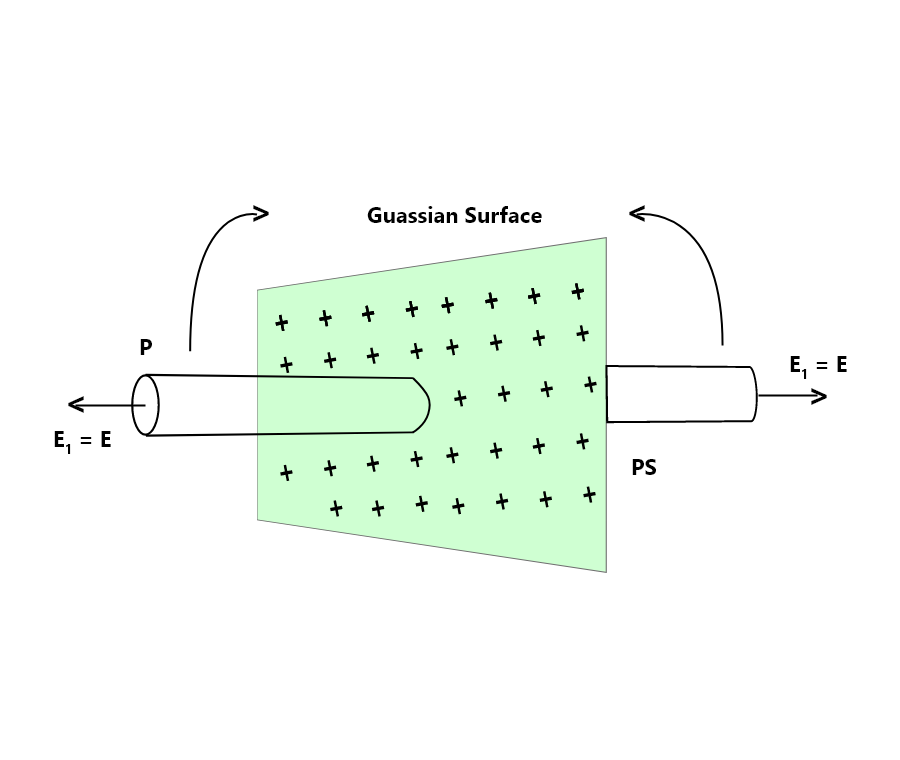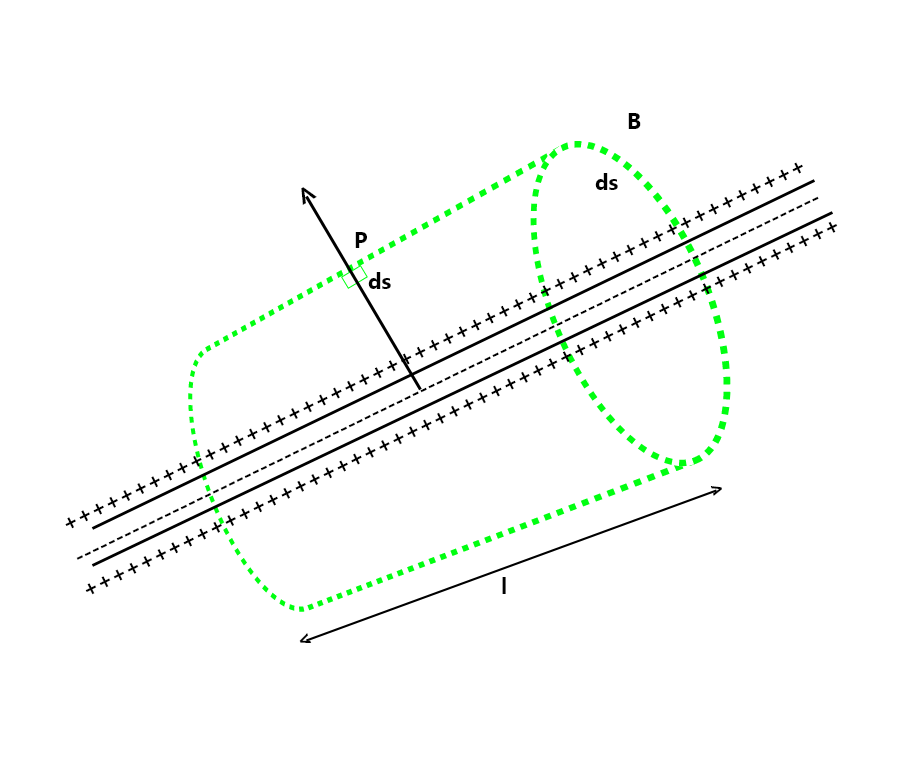# Electric Field due to Uniformly Charged Infinite Plane Sheet and Thin Spherical Shell

• Last Updated : 25 Mar, 2022

The study of electric charges at rest is the subject of electrostatics. By forming an electric field, the electrical charge affects the properties of the surrounding environment. The electric field is a property of a charging system. Karl Friedrich Gauss (1777-1855), one of the greatest mathematicians of all time, developed Gauss’ law, which expresses the connection between electric charge and electric field. In that, it represents the link between electric field and electric charge, Gauss’ law is equivalent to Coulomb’s law. Gauss’ law gives a comparable approach for determining electric intensity expressions.

### What is Gauss’s law?

Gauss Law, often known as Gauss’ flux theorem or Gauss’ theorem, is the law that describes the relationship between electric charge distribution and the consequent electric field.

According to Gauss’s law, the total quantity of electric flux travelling through any closed surface is proportional to the contained electric charge. The electrical field of a surface is determined using Coulomb’s equation, but the Gauss law is necessary to calculate the distribution of the electrical field on a closed surface. It describes the electrical charge contained inside the closed surface or the electrical charge existing within the enclosed closed surface.

The total flux contained within a closed surface equals 1/ε0 times the total electric charge enclosed by the closed surface, according to Gauss Law.

The total charge enclosed in a closed surface is proportional to the total flux enclosed by the surface, according to the Gauss theorem. Therefore, if φ is total flux and ε0 is electric constant, the total electric charge Q enclosed by the surface is,

Q = φ ε0

Hence, the Gauss law formula is expressed in terms of charge as,

φ = Q / ε0

where,

• Q is total charge within the given surface, and
• ε0 is the electric constant.

The net flow through a closed surface is proportional to the net charge in the volume surrounded by the closed surface.### Electric Field due to Uniformly Charged Infinite Plane SheetThe electric field generated by the infinite charge sheet will be perpendicular to the sheet’s plane. Consider a cylindrical Gaussian surface whose axis is perpendicular to the sheet’s plane. Gauss’s Law may be used to calculate the electric field.

According to Gauss’s Law:

φ = Q / ε0      …..(1)

Charge q will be σA as a result of continuous charge distribution. We shall only consider electric flow from the two ends of the hypothetical Gaussian surface when discussing net electric flux. This is due to the fact that the curved surface area and the electric field are perpendicular to each other, resulting in zero electric flux. As a result, the net electric flow will be:

φ = EA – (-EA) = 2EA

Therefore, from equation (1):

2EA = Q / ε0

On rearranging for E as,

E = Q / 2ε0

### Electric Field due to Uniformly Charged Infinite Plane SheetConsider the radius “R” and the thin spherical shell of the density of the surface charge. The shell exhibits spherical symmetry, as may be seen by observing it. The electric field produced by the spherical shell can be measured in two ways:

• Electric Field Outside the Spherical Shell
• Electric Field Inside the Spherical Shell

Electric Field Outside the Spherical Shell:

Consider a point P outside the spherical shell at a distance r from the centre of the spherical shell to determine an electric field outside the shell. We use a Gaussian spherical surface with radius r and centre O for symmetry. Because all points are equally spaced “r” from the sphere’s centre, the Gaussian surface will pass through P and experience a constant electric field all around. Then, according to Gauss’s law:

φ = q / ε0

Since a charge is enclosed inside the spherical Gaussian surface q, which is equal to σ × 4 πR2. Therefore,

φ = σ × 4 πR2 / ε0         …..(1)

The total electric flux through the Gaussian surface will be:

φ = E × 4 πr2

So from equation (1):

σ × 4 πR2 / ε0 = E × 4 πr2

or

E = σ R2 / ε0 r2

Since, the surface charge density, σ is q / 4 πR2

E = kq / r2

Electric Field Inside the Spherical Shell:

To find the electric field inside the spherical shell, consider a point P inside the shell. We pick the spherical Gaussian surface travelling through P, centred at O, and radius r by symmetry. Now, according to Gauss’ law,

φ = q / ε0

Since the total electric flux inside the Gaussian surface will be:

φ = E × 4 πr2 = 0

Hence,

E = 0

### Sample Problems

Problem 1: A thin long cylinder of radius 1 cm carrying a charge of 5 μC/m is kept in water. Find the electric field intensity at a point situated at a distance of 10 cm from the axis of the cylinder if it is immersed in water. (kwater = 81)

Solution:

Given that,

q = 5 μC/m = 5 × 10-6 C/m

R = 1 cm = 10-2 m

r = 10 m = 10-1 m

kwater = 81

The Electric field intensity at a point outside charged conducting cylinder is,

E = q / 2πkε0 r

= 2q / 4πε0 r

= (1 / 4πε0) × q / kr

= 9 × 109 × (2 × 5 × 10-6 / 81 × 10-1)

= 1.111 × 104 N/C

Problem 2: A long cylinder of radius 2 cm carries a charge of 5 µC/m kept in a medium of dielectric constant 10. Find the electric field intensity at a point situated at a distance of 1 m from the axis of the cylinder.

Solution:

Given that,

R = 2 cm

σ = 5 μC/m

k =10

r = 1 m

Since,

E = (1 / 4πε0) × q / kr

=  9 × 109 × (2 × 5 × 10-6 / 10 × 1)

= 9 × 103 V/m

Problem 3: A large plane sheet of charge having surface charge density 5 × 10-6 C / m2) lies in the air. Find electric field intensity near the sheet.

Solution:

Given that,

σ = 5 × 10-6 C/m2

ε0 = 8.85 × 10-2 C2 /Nm2

k = 1

Electric field intensity near the sheet is,

E = σ / 2kε0

= 5 × 10-6 / (2  × 1 × 8.85 × 10-12)

= 2.824 × 105 N/C

Problem 4: A uniformly charged cylinder of length 10 cm has a charge of one microcoulomb. If it is in a medium of dielectric constant 5, find the intensity at a point outside the cylinder. The distance of the point from the axis of the cylinder equals its length.

Solution:

Given that,

ε0 = 8.85 × 10-12 C2 / Nm2

l = 10cm = 0.1m

Q = 1μC = 10-6 C

k = 5

r = l = 0.1m

Linear charge density is,

q = Q / l

= 10-6 / 0.1

= 10-5 C/m

Electric field intensity is,

E = (1 / 4πε0) × q / kr

= 9 × 109 × (2 × 10-5 / 5 × 10-1)

= 3.6 × 105 V/m

Problem 5: Find the surface charge of a large plane sheet of charge having electric field intensity near the sheet of 2.8 × 105 N/C, kept in the air. (kair = 1)

Solution:

Given that,

kair = 1

E = 2.8 ×  105 N/C

ε0 = 8.85 × 10-2 C2 /Nm2

E = σ / 2kε0

2.8 × 105 N/C = σ × (2 × 8.85 × 10-2)

= 4.95 × 10-6 C /m2

My Personal Notes arrow_drop_up
Recommended Articles
Page :